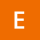Home Communities
IT Knowledge
Inspiration
Languages
EN

# Java - convert int to long

5 points
Created by:Evie-Grace-Noble
351

## Short solutions

``````// from int
int number = 200;

// 1. int to long
long result = (long) number;
// 2. int to long
long result = 1L * number;
// 3. int to Long
Long result = Long.valueOf(number);

// from Integer
Integer number = 200;

// 1. Integer to long
long result = number.longValue();
// 2. Integer to Long
Long result = Long.valueOf(number);``````

Below we have full examples of how to convert int to long.

## 1. Using explicit long casting

``````public class Example1 {

public static void main(String[] args) {

int number = 200;
long longNumber = (long) number;

longNumber += 5L * Integer.MAX_VALUE;
System.out.println(longNumber); // 10737418435
}
}``````

Output:

``10737418435``

## 2. Using implicit long casting

``````public class Example2 {

public static void main(String[] args) {

int number = 200;
long longNumber = number;

longNumber += 5L * Integer.MAX_VALUE;
System.out.println(longNumber); // 10737418435
}
}``````

Output:

``10737418435``

## 3. Using multiplication by long number

``````public class Example3 {

public static void main(String[] args) {

int number = 200;
long longNumber = 1L * number;

longNumber += 5L * Integer.MAX_VALUE;
System.out.println(longNumber); // 10737418435
}
}``````

Output:

``10737418435``

## 4. Convert to int to Long using Long constructor

``````public class Example4 {

public static void main(String[] args) {

int number = 200;
Long longNumber = new Long(number);

longNumber += 5L * Integer.MAX_VALUE;
System.out.println(longNumber); // 10737418435
}
}``````

Output:

``10737418435``

Note:
Syntax of Long constructor:
`public Long(long value)`

As we can see this constructor get long as parameter, so in our case we just made casting from int to long.

When we see the hint from intellij idea, we will see 'Remove boxing' and when we apply it, our code will convert
from:
`Long longNumber = new Long(number);`

to:
`Long longNumber = (long) number;`

When we use new Long constructor with int parameter we get hint from intellij idea:

Unnecessary boxing 'Long.valueOf(number)' less... (Ctrl+F1)
Inspection info: Reports explicit boxing, i.e. wrapping of primitive values in objects. Explicit manual boxing is unnecessary under Java 5 and newer, and can be safely removed.
This inspection only reports if the project or module is configured to use a language level of 5.0 or higher.

## 5. Convert to int to Long using Long.valueOf()

``````public class Example5 {

public static void main(String[] args) {

int number = 200;
Long longNumber = Long.valueOf(number);

longNumber += 5L * Integer.MAX_VALUE;
System.out.println(longNumber); // 10737418435
}
}``````

Output:

``10737418435``

Note:
The same hint from intellij idea as for Long constructor.

Syntax of Long.valueOf() method:
`public static Long valueOf(long l)`

Intellij idea, hint 'Remove boxing' will convert
from:
`Long longNumber = Long.valueOf(number);`

to:
`Long longNumber = (long) number;`

When we use Long.valueOf() we get hint from intellij idea:
Unnecessary boxing 'Long.valueOf(number)' less... (Ctrl+F1)

## 6. Convert Integer to Long using Integer longValue() method

``````public class Example6 {

public static void main(String[] args) {

Integer number = 200;
long longNumber = number.longValue();

longNumber += 5L * Integer.MAX_VALUE;
System.out.println(longNumber); //  10737418435
}
}``````

Output:

``10737418435``